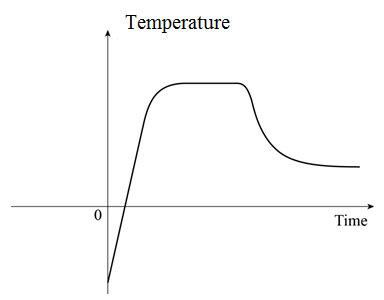# To plot: The graph of the temperature of the pie as a function of time.### Precalculus: Mathematics for Calcu...

6th Edition
Stewart + 5 others
Publisher: Cengage Learning
ISBN: 9780840068071### Precalculus: Mathematics for Calcu...

6th Edition
Stewart + 5 others
Publisher: Cengage Learning
ISBN: 9780840068071

#### Solutions

Chapter 2.1, Problem 81E
To determine

## To plot: The graph of the temperature of the pie as a function of time.

Expert Solution

### Explanation of Solution

The temperature of pie starts below the 0 due to frozen state and next the temperature increases and levels off due to bake in oven for 1 hour and finally the temperature cools down before eating or consumption.Figure (1)

Figure (1) shows the graph of temperature of pie as function of time.

### Have a homework question?

Subscribe to bartleby learn! Ask subject matter experts 30 homework questions each month. Plus, you’ll have access to millions of step-by-step textbook answers!